Normal view MARC view ISBD view

Introductory econometrics : using Monte Carlo simulation with Microsoft Excel / Humberto Barreto, Frank M. Howland.

Material type:TextPublisher: Cambridge ; New York : Cambridge University Press, 2006Description: xxiii, 774 p. : ill. ; 26 cm.ISBN: 9780521843195; 0521843197.DDC classification: 330.01518282 Online resources: Worldcat details | Ebook Fulltext
Contents:
1. Introduction; Part I. Description: 2. Correlation; 3. Pivot tables; 4. Computing regression; 5. Interpreting regression; 6. Functional form; 7. Multivariate regression; 8. Dummy variables; Part II. Inference: 9. Monte Carlo simulation; 10. Inferential statistics review; 11. Measurement box model; 12. Comparing two populations; 13. The classical econometric model; 14. The Gauss Markov theorem; 15. Understanding the standard error; 16. Hypothesis testing and confidence intervals; 17. F tests; 18. Omitted variable bias; 19. Heteroskedasticity; 20. Autocorrelation; 21. The series topics; 22. Dummy dependent variables; 23. Bootstrap; 24. Simultaneous equations. Table of contents
Tags from this library: No tags from this library for this title.Average rating: 0.0 (0 votes)
Item type Current location Collection Call number Copy number Status Date due Barcode Item holdsE-Book
E-book
Non-fiction 330.01518282 BAI 2006 (Browse shelf) Not for loanText
Reserve Section
Non-fiction 330.01518282 BAI 2006 (Browse shelf) C-1 Not For Loan 19627CDs & DVDs
Audio Visual
Non-fiction 330.01518282 BAI 2006 (Browse shelf) C-1 Available CD-1165
Total holds: 0
Browsing EWU Library Shelves , Shelving location: E-book Close shelf browser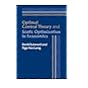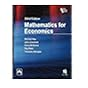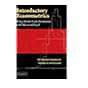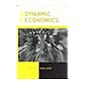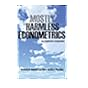330.0151 LEO 1992 Optimal control theory and static optimization in economics / 330.0151 MAT 2006 Mathematics for economics / 330.0151 MAT 2011 Mathematics for economics / 330.01518282 BAI 2006 Introductory econometrics : 330.015193 VEE 2003 Economics and the theory of games / 330.015195 ADD 2003 Dynamic economics : 330.015195 ANM 2009 Mostly harmless econometrics :

Includes bibliographical references and index.

1. Introduction; Part I. Description: 2. Correlation; 3. Pivot tables; 4. Computing regression; 5. Interpreting regression; 6. Functional form; 7. Multivariate regression; 8. Dummy variables; Part II. Inference: 9. Monte Carlo simulation; 10. Inferential statistics review; 11. Measurement box model; 12. Comparing two populations; 13. The classical econometric model; 14. The Gauss Markov theorem; 15. Understanding the standard error; 16. Hypothesis testing and confidence intervals; 17. F tests; 18. Omitted variable bias; 19. Heteroskedasticity; 20. Autocorrelation; 21. The series topics; 22. Dummy dependent variables; 23. Bootstrap; 24. Simultaneous equations. Table of contents

Economics

There are no comments for this item.